# Candy and boxes

We have some candy and empty boxes. When we put candies in boxes of ten, there will be 2 candies and 8 empty boxes left. When of eight, there will be 6 candies and 3 boxes left. How many candy and empty boxes left when we put candies in boxes of nine?

x =  51
y =  11

### Step-by-step explanation: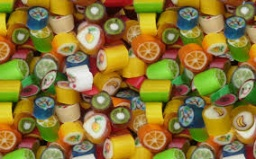Did you find an error or inaccuracy? Feel free to write us. Thank you!

Showing 1 comment:Math student
this didnt  help meTips to related online calculators
Do you have a linear equation or system of equations and looking for its solution? Or do you have a quadratic equation?
Do you solve Diofant problems and looking for a calculator of Diofant integer equations?
Do you want to perform natural numbers division - find the quotient and remainder?

## Related math problems and questions:

• Columns of two and threeWhen students in one class stand in columns of two, there is none left. When he stands in columns of three, there is one student left. There are 5 more double columns than three columns. How many students are in the class?
• CandiesIf Alena give Lenka 3 candy will still have 1 more candy. If Lenka give Alena 1 candy Alena will hame twice more than Lenka. How many candies have each of them?
• GrandmotherGrandmother wants to give the candies to grandchildren that when she gives 5 candy everyone 3 missing and when she gives 4 candies 3 is surplus. How many grandchildren has grandmother and how many candies has?
• Sweets, candyGrandfather gave out sweets to four children. At the last moment, two more children came, so in order to have them all the same, each of the four children would receive four candies less than they would have received if they had not. How much did my grand
• 600 pencils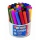600 pencils we want to be divided into three groups. The biggest groups have ten pens more than the smallest. How many ways can this be done?
• Three numbersWe have three different non-zero digits. We will create all 3 digits numbers from them to use all 3 figures in each number. We add all the created numbers, and we get the sum of 1554. What were the numbers?
• By sixFrom the digits 1,2,3,4 we create the long integer number 123412341234. .. .. , which will have 962 digits. Is this number divisible by 6?
• Diofant equationIn the set of integers (Z) solve the equation: 212x +316y =0 Write result with integer parameter t in Z (parameter t = ...-2,-1,0,1,2,3... if equation has infinitely many solutions)
• AntennasIf you give me two antennas will be same. If you give me again your two antenna I have a 5× so many than you. How many antennas have both mans?
• RemainderA is an arbitrary integer that gives remainder 1 in the division with 6. B is an arbitrary integer that gives remainder 2 the division by. What makes remainder in division by 3 product of numbers A x B ?
• DivisibilityIs the number 761082 exactly divisible by 9? (the result is the integer and/or remainder is zero)
• Balls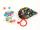Michal said to Martin: give me one ball, and I'll have twice as you. Martin said: give me 4, and we will have equally. How many balls each have?
• Two integers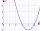Two integers, a and b, have a product of 36. What is the least possible sum of a and b?
• Two-digit numberIn a two-digit number, the number of tens is three greater than the number of units. If we multiply the original number by a number written in the same digits but in reverse order, we get product 3 478. Find the original number.
• SchoolLess than 500 pupils attend school. When it is sorted into pairs, one pupil remains. Similarly, when sorted into 3, 4, 5 and 6 members team one remains. Sorted to seven members teams, no left behind. How many pupils are attending this school?
• Three digits numberFrom the numbers 1, 2, 3, 4, 5 create three-digit numbers that digits not repeat and number is divisible by 2. How many numbers are there?
• 1200 screws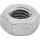1200 screws are to be divided into 3 groups so that in the 1st group there are 300 screws more than in the 2nd group and in the 2nd group 150 screws less than in the 3rd group. How many screws will be in each group?